# Difference Between Electron Geometry and Molecular Geometry

## Main Difference – Electron Geometry vs Molecular Geometry

The geometry of a molecule determines the reactivity, polarity and biological activity of that molecule. The geometry of a molecule can be given as either the electron geometry or the molecular geometry. The VSEPR theory (Valence Shell Electron Pair Repulsion theory) can be used to determine the geometries of molecules. Electron geometry includes the lone electron pairs present in a molecule. Molecular geometry can be determined by the number of bonds that a particular molecule has. The main difference between electron geometry and molecular geometry is that electron geometry is found by taking both lone electron pairs and bonds in a molecule whereas molecular geometry is found using only the bonds present in the molecule.

### Key Areas Covered

1. What is Electron Geometry
– Definition, Identification, Examples
2. What is Molecular Geometry
– Definition, Identification, Examples
3. What are Geometries of Molecules
– Explanatory Chart
4. What is the Difference Between Electron Geometry and Molecular Geometry
– Comparison of Key Differences

Key Terms: Electron Geometry, Lone Electron Pair, Molecular Geometry, VSEPR Theory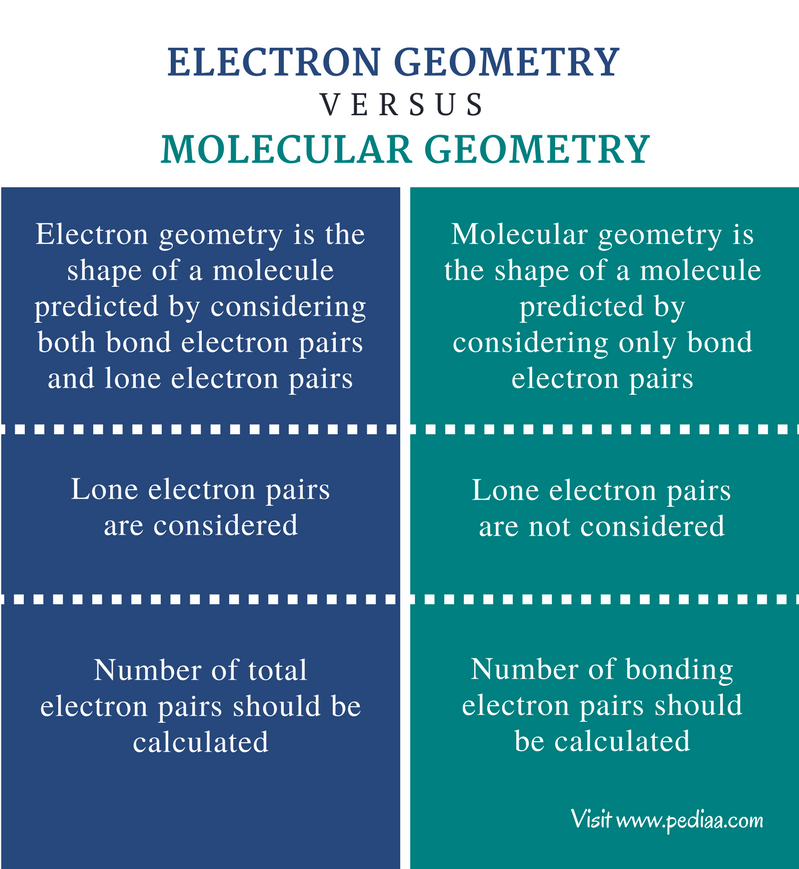## What is Electron Geometry

Electron geometry is the shape of a molecule predicted by considering both bond electron pairs and lone electron pairs. The VSEPR theory states that electron pairs located around a certain atom repel each other. These electron pairs can be either bonding electrons or non-bonding electrons.

The electron geometry gives the spatial arrangement of all the bonds and lone pairs of a molecule. The electron geometry can be obtained using VSEPR theory.

### How to Determine Electron Geometry

The following are the steps used in this determination.

1. Predict the central atom of the molecule. It should be the most electronegative atom.
2. Determine the number of valence electrons in the central atom.
3. Determine the number of electrons donated by other atoms.
4. Calculate the total number electrons around the central atom.
5. Divide that number from 2. This gives the number of electron groups present.
6. Deduct the number of single bonds present around the central atom from the steric number obtained above. This gives the number of lone electron pairs present in the molecule.
7. Determine the electron geometry.

#### Electron Geometry of CH4

Central atom of the molecule                                       =     C

Number of valence electrons of C                                =     4

Number of electrons donated by hydrogen atoms   =   4 x (H)
=   4 x 1   =  4

Total number of electrons around C                           =   4 + 4    =    8

Number of electron groups                                           =  8 / 2     =     4

Number of single bonds present                                  =  4

Number of lone electron pairs                                      =  4 – 4     = 0

Therefore, the electron geometry                                  =   tetrahedral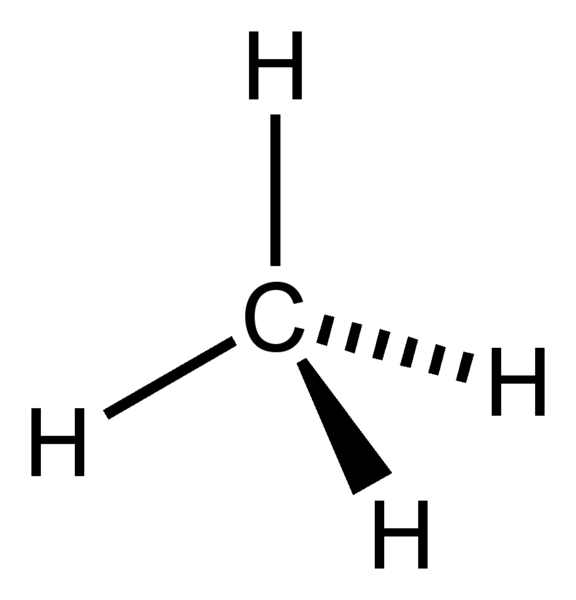Figure 1: Electron Geometry of CH4

#### Electron Geometry of Ammonia (NH3)

Central atom of the molecule                                         = N

Number of valence electrons of N                                 = 5

Number of electrons donated by hydrogen atoms     = 3 x (H)
= 3 x 1 = 3

Total number of electrons around N                            = 5 + 3 = 8

Number of electron groups                                             = 8 / 2 = 4

Number of single bonds present                                    = 3

Number of lone electron pairs                                        = 4 – 3 = 1

Therefore, the electron geometry                                   = tetrahedral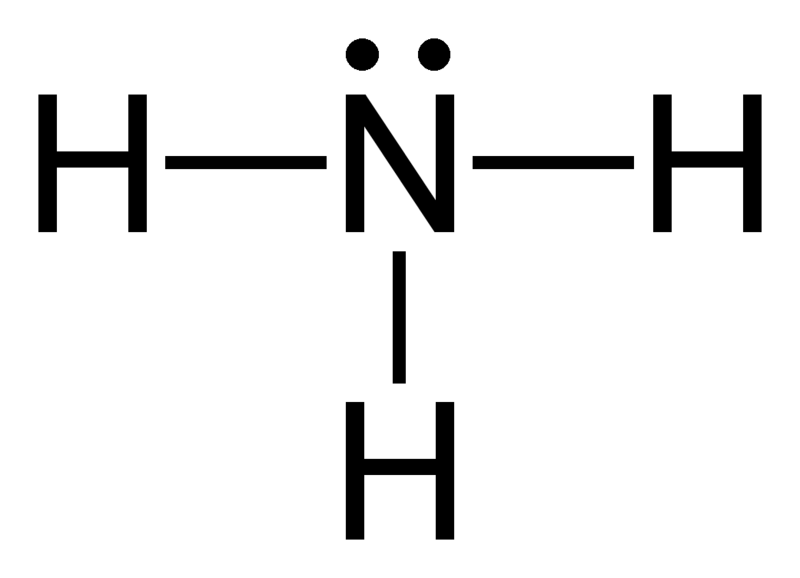Figure 2: Electron Geometry of Ammonia

#### Electron Geometry of AlCl3

Central atom of the molecule                                        = Al

Number of valence electrons of Al                               = 3

Number of electrons donated by Cl atoms                 = 3 x (Cl)
= 3 x 1 = 3

Total number of electrons around N                           = 3 + 3 = 6

Number of electron groups                                           = 6 / 2 = 3

Number of single bonds present                                  = 3

Number of lone electron pairs                                      = 3 – 3 = 0

Therefore, the electron geometry                                 = trigonal planar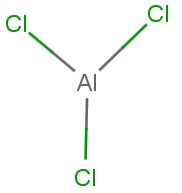Figure 3: Electron Geometry of AlCl3

Sometimes, the electron geometry and the molecular geometry are the same. That is because only bonding electrons are considered in the determination of geometry in the absence of lone electron pairs.

## What is Molecular Geometry

Molecular geometry is the shape of a molecule predicted by considering only bond electron pairs. In this case, lone electron pairs are not taken into account. Moreover, double bonds and triple bonds are considered as single bonds. The geometries are determined based on the fact that lone electron pairs need more space than bonding electron pairs. For example, if a certain molecule is composed of two pairs of bonding electrons along with a lone pair, the molecular geometry is not linear. The geometry there is “bent or angular” because the lone electron pair needs more space than two bonding electron pair.

### Examples of Molecular Geometry

#### Molecular Geometry of H2O

Central atom of the molecule                                       = O

Number of valence electrons of O                               = 6

Number of electrons donated by hydrogen atoms  = 2 x (H)
= 2 x 1 = 2

Total number of electrons around N                         = 6 + 2 = 8

Number of electron groups                                         = 8 / 2 = 4

Number of lone electron pairs                                    = 2

Number of single bonds present                                = 4 – 2 = 2

Therefore, electron geometry                                     = Bent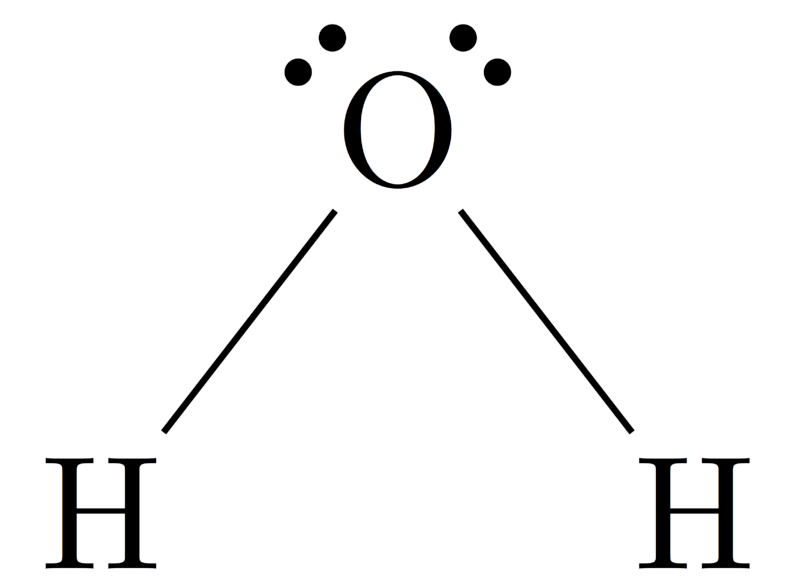Figure 4: Molecular geometry of H2O

#### Molecular Geometry of Ammonia (NH3)

Central atom of the molecule                                        = N

Number of valence electrons of N                                = 5

Number of electrons donated by hydrogen atoms   = 3 x (H)
= 3 x 1 = 3

Total number of electrons around N                           = 5 + 3 = 8

Number of electron groups                                           = 8 / 2 = 4

Number of lone electron pairs                                      = 1

Number of single bonds present                                  = 4 – 1 = 3

Therefore, electron geometry                                        = trigonal pyramid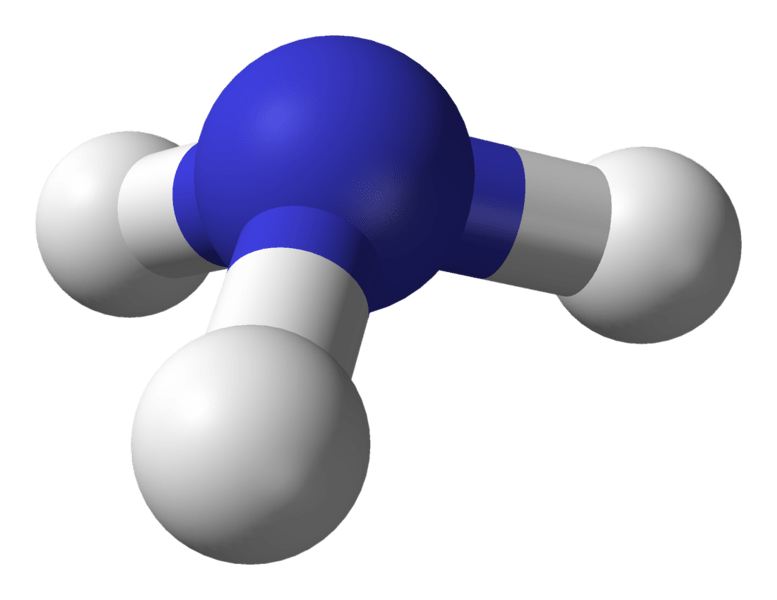Figure 5: Ball and stick structure for ammonia molecule

The electron geometry of ammonia is tetrahedral. But the molecular geometry of ammonia is trigonal pyramid.

## Geometry of Molecules

The following chart shows some geometries of molecules according to the number of electron pairs present.

 Number of electron pairs Number of bonding electron pairs Number of lone electron pairs Electron geometry Molecular geometry 2 2 0 Linear Linear 3 3 0 Trigonal planar Trigonal planar 3 2 1 Trigonal planar Bent 4 4 0 Tetrahedral Tetrahedral 4 3 1 Tetrahedral Trigonal pyramid 4 2 2 Tetrahedral Bent 5 5 0 Trigonal bypyramidal Trigonal bypyramidal 5 4 1 Trigonal bypyramidal Seesaw 5 3 2 Trigonal bypyramidal T-shaped 5 2 3 Trigonal bypyramidal Linear 6 6 0 Octahedral OctahedralFigure 6: Basic Geometries of Molecules

The above table shows basic geometries of molecules. The first column of geometries shows electron geometries. Other columns show molecular geometries including the first column.

## Difference Between Electron Geometry and Molecular Geometry

### Definition

Electron Geometry: Electron geometry is the shape of a molecule predicted by considering both bond electron pairs and lone electron pairs.

Molecular Geometry: Molecular geometry is the shape of a molecule predicted by considering only bond electron pairs.

### Lone Electron Pairs

Electron Geometry: Lone electron pairs are considered when finding the electron geometry.

Molecular Geometry: Lone electron pairs are not considered when finding the molecular geometry.

### Number of Electron Pairs

Electron Geometry: The number of total electron pairs should be calculated to find the electron geometry.

Molecular Geometry: The number of bonding electron pairs should be calculated to find the molecular geometry.

### Conclusion

Electron geometry and molecular geometry are the same when there are no lone electron pairs on the central atom. But if there are lone electron pairs on the central atom, the electron geometry always differ from the molecular geometry. Therefore, the difference between electron geometry and molecular geometry depends on lone electron pairs present in a molecule.

##### References:

1. “Molecular Geometry”. N.p., n.d. Web. Available here. 27 July 2017.
2.”VSEPR theory.” Wikipedia. Wikimedia Foundation, 24 July 2017. Web. Available here.  27 July 2017.

##### Image Courtesy:

1. “Methane-2D-small”  (Public Domain) via Commons Wikimedia
2. “Ammonia-2D-flat” By Benjah-bmm27 – Own work (Public Domain) via Commons Wikimedia
3. “AlCl3” By Dailly Anthony – Own work (CC BY-SA 3.0) via Commons Wikimedia
4. “H2O Lewis Structure PNG” By Daviewales – Own work (CC BY-SA 4.0) via Commons Wikimedia
5. “Ammonia-3D-balls-A” By Ben Mills – Own work (Public Domain) via Commons Wikimedia
6. “VSEPR geometries” By Dr. Regina Frey, Washington University in St. Louis – Own work, Public Domain) via Commons Wikimedia